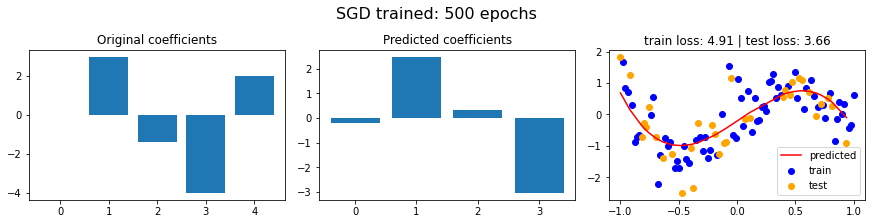import tensorflow as tf
import matplotlib.pyplot as plt
import numpy as np
from sklearn.model_selection import train_test_split
import pandas as pd
from tqdm import tqdm


## Make training data

def poly(x, coeffs): return np.sum([coeff*np.power(x, i) for i, coeff in enumerate(coeffs)], axis=0)


Make a Pluto notebook version of this where the coeffs are scrubbables, could also use Ipywidgets here as well

coeffs = [0, 3, -1.4, -4, 2]
sigma = 0.6

X = np.linspace(-1, 1, 100)
y = poly(X, coeffs) + sigma*np.random.randn(*X.shape)

plt.scatter(X, y)

<matplotlib.collections.PathCollection at 0x7f5181c46350>X_train, X_test, y_train, y_test = train_test_split(X, y, test_size=0.33, random_state=42)
list(map(np.shape, [X_train, X_test, y_train, y_test]))

[(67,), (33,), (67,), (33,)]
def sort(x,y ):
df = pd.DataFrame({'x': x, 'y': y})
df.sort_values(by='x', inplace=True)
return df['x'], df['y']

X_train, y_train = sort(X_train, y_train)
X_test, y_test = sort(X_test, y_test)


## Baseline: Linear Algebra method $\rightarrow$ vandermonde solution

Nparams = 4
A = np.vander(X_train, N=Nparams, increasing=True)
c, yerr, _, _ = np.linalg.lstsq(A, y_train, rcond=None)
print(f'MSE error: {yerr**2/len(y_train):0.2f}')

MSE error: 8.12

def vander_model(x, w):
A = np.vander(x, N=len(w), increasing=True)
return A@w

def get_model_losses(model):
train_loss = np.linalg.norm(model(X_train) - y_train, ord=2)
test_loss = np.linalg.norm(model(X_test) - y_test, ord=2)
return train_loss, test_loss

train_loss, test_loss = get_model_losses(lambda x: vander_model(x, c))

print(f'test loss: {test_loss:0.2f}')
print(f'train loss: {train_loss:0.2f}')

test loss: 3.44
train loss: 4.83

def eval_model(model, params, name=''):
f, axs = plt.subplots(1,3, figsize=(12,3), constrained_layout=True)

f.suptitle(name, fontsize=16)
axs.bar(range(len(coeffs)), coeffs)
axs.set_title('Original coefficients')
axs.bar(range(len(params)), params)
axs.set_title('Predicted coefficients')
axs.scatter(X_train, y_train, color='blue', label='train')
axs.scatter(X_test, y_test, color='orange', label='test')
axs.plot(X_test, model(X_test), 'r', label='predicted')

train_loss, test_loss = get_model_losses(model)

axs.set_title(f'train loss: {train_loss:0.2f} | test loss: {test_loss:0.2f}')
axs.legend()

eval_model(lambda x: vander_model(x, c), c, 'Vandermonde')## Tensorflow SGD

Nparams = 4
np.random.seed(42)
params = tf.Variable(tf.constant(np.random.rand(1, Nparams)), trainable=True, name='weights')

def model(x, w):
return tf.reduce_sum([p*tf.pow(x, i) for i, p in enumerate(w)], axis=0)

eval_model(lambda x: model(x, params), *params.numpy(), 'initial random state')lr = 0.1
nepochs = 500
cost = lambda: tf.losses.mse(y_train, model(X_train, params))
optim = tf.keras.optimizers.SGD(learning_rate=lr)

for _ in tqdm(range(nepochs)):
optim.minimize(cost, params)

100%|██████████| 500/500 [00:03<00:00, 159.58it/s]

eval_model(lambda x: model(x, params), *params.numpy(), f'SGD trained: {nepochs} epochs')## Conclusions

This contrived situation is very easy to solve. I suspect Chris Mattman's NYC 311 is much more challenging because most values are zero and thus the gradients can easily go to zero if there is poor initialization

Tensorflow basic regression tutorial